Study of mathematics online.
Study math with us and make sure that "Mathematics is easy!"

# Area Formulas for Geometric Figures

## Area of a triangle formulas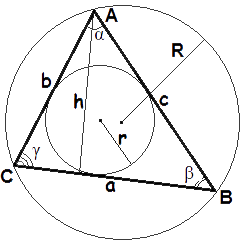1. Area of a triangle when we know the base and the height

The area of a triangle is equal to half of base times height.

 A = 1 a · h 2
2. Area of a triangle when we know the lengths of all three of its sides

### Heron's formula

A = √s(s - a)(s - b)(s - c)
3. Area of a triangle when we know two sides and the included angle

The area of a triangle is equal to half of a product of two sides and sine of the angle between this sides.

 A = 1 a · b · sin γ 2
 A = 1 a · c · sin β 2
 A = 1 b · c · sin α 2
4. Area of a triangle when we know three sides and circumradius
 A = a · b · с 4R
5. Area of a triangle when we know semiperimeter and in-radius

The area of a triangle is equal to semiperimeter times in-radius.

 A = s · r
where A - the area of a triangle,
a, b, c - the length of sides BC,AC,AB accordingly,
h - the height, the length of the altitude,
α, β, γ - the angles,
r - the length of the in-radius,
R - the length of the circumradius,
 s = a + b + c - the semiperimeter, or half of the triangle's perimeter. 2

## Area of a square formulas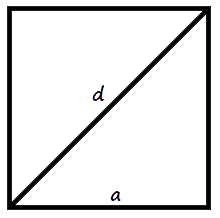1. Area of a square when we know the length of a side

The area of a square is equal to squared side.

A = a2
2. Area of a square when we know the length of diagonal

The area of a square is half of squared diagonal.

 A = 1 d2 2
where A - the area of a square,
a - the lenth of side,
d - the length of diagonal.

## Area of a rectangle formula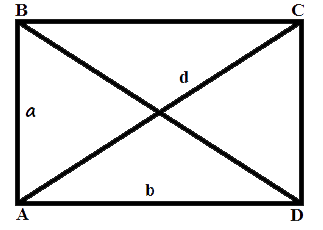The area of a rectangle is equal to a product of lenghts of two sides (height and width)
A = a · b
where A - the area of a rectangle,
a, b - the length of sides(the height and width of rectangle).

## Area of a parallelogram formulas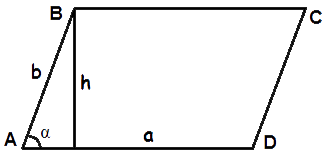1. Area of a parallelogram when we know the side and the height

The area of a parallelogram is equal to side times height.

A = a · h
2. Area of a parallelogram when we know two sides and the included angle

The area of a parallelogram is equal to a product of two sides and sine of the angle between this sides.

A = a · b · sin α
3. where A - the area of a parallelogram,
a, b - lengths of sides,
h - the height, the length of the altitude,
α - the measure of the angle between sides.

## Area of a rhombus formulas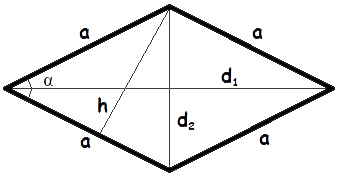1. Area of a rhombus when we know the side and the height

The area of a rhombus is equal to side times height.

A = a · h
2. Area of a rhombus when we know length of a side and the included angle

The area of a rhombus is equal to a product of the squared side and sine of the angle between sides of a rhombus.

A = a2 · sin α
3. Area of a rhombus when we know length of its diagonals

The area of a rhombus is equal to half a product of it diagonals lengths.

 A = 1 d1 · d2 2
where A - the area of a rhombus,
a - the length of a side,
h - the height, the length of the altitude,
α - the measure of the angle between sides of a rhombus,
d1, d2 - the length of diagonals.

## Area of a trapezium formulas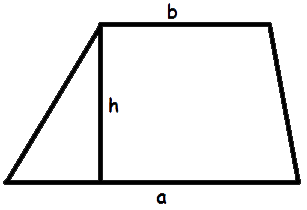1. Heron's formula for a trapezium
 A = a + b √(s-a)(s-b)(s-a-c)(s-a-d) |a - b|
2. Area of a trapezium when we know length of 2 bases and the height

The area of a trapezium is equal to product of the height and half-sum of two bases

 A = 1 (a + b) · h 2
where A - the area of a trapezium,
a, b - the length of the 2 bases,i.e., the parallel sides,
c, d - length of the legs (the lateral sides),
 s = a + b + c + d - the semiperimeter, or half of the trapezium's perimeter. 2

## Area of a quadrangle formulas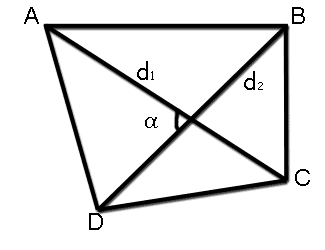1. Area of a quadrangle when we know length of its diagonals and angle between diagonals

The area of a quadrangle is equal to product of its diagonals and and sine of the angle between diagonals

 A = 1 d1 d2 sin α 2

where A - the area of a quadrangle,
d1, d2 - the length of diagonals,
α - the angle between diagonals.

2. Area of a quadrangle when we know length of its perimeter and in-radius

A = s · r
3.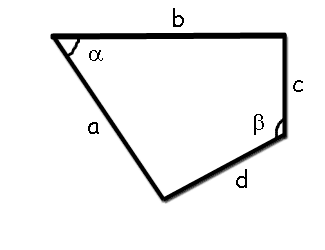Area of a quadrangle when we know length of its sides and value of opposite corners
A = √(s - a)(s - b)(s - c)(s - d) - abcd cos2θ
where A -the area of a quadrangle,
a, b, c, d - the length of sides,
 s = a + b + c + d - semiperimeter of quadrangle, 2
 θ = α + β - half the sum of two opposite angles of a quadrilateral. 2

## Area of a circle formulas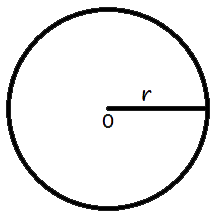1. Area of a circle when we know its radius

The area of a circle is equal to a product of squared radius and pi.

A = π r2
2. Area of a circle when we know its diameter

The area of a circle is equal to a quarter product of squared diameter and pi.

 A = 1 π d2 4
where A - the area of a circle,
r - the length of the radius,
d - the length of the diameter.

## Area of a ellipse formulas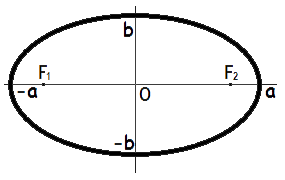The area of an ellipse is equal to a product of lengths of the major and minor semiaxes and pi.
A = π · a · b
where A - the area of an ellipse,
a - the length of the major semiaxis,
b - the length of the minor semiaxis,